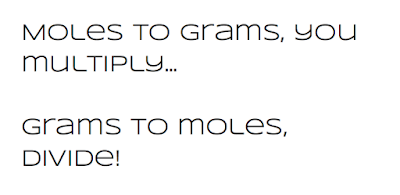## Wednesday, November 16, 2016

### Balancing Equations and Conservation of Matter

The action of balancing chemical equations primarily sets out to find the ratios of compounds that result in there being the same number of atoms in the product as there were in the reactants. This is the most fundamental concept that must be understood. It is so important, it bears being repeated and emphasized.

At the most basic level, balancing chemical equations is the process of finding coefficients which result in the number of atoms in the reactants being equal to the number of atoms in the product.

If there are 8 atoms total in all of the molecules of the reactants, then there MUST be 8 atoms total in all of the molecules of the products.

It does not matter how many different compounds are involved. It does not matter how many molecules of any of the compounds are involved. The driving factor in balancing equations is that the number of atoms on each side of the reaction are equal.

Read that last sentence ten times!

Conservation of Matter

The reason that the number of atoms must be the same stems from the Law of Conservation of Matter. In basic terms, the Law of Conservation states that matter cannot be created or destroyed. It really, if thought about, is logical. If you have 15 atoms of something, no matter how you combine or arrange them, you end up with 15 of something.

The CRINGE has NO LIMITS

This law drives chemistry and chemical reactions. This is why that the number of atoms cannot change.

One way to look at this principle as it applies to chemical reactions is to compare the mass of the reactants to the mass of the products. Because the number of atoms CANNOT change, and because each atom has a certain mass, there should be no difference between the mass of the products and the mass of the reactants.

Consider the reaction of hydrogen and oxygen as an example:

2H2 + O2 = 2H2O

This reaction begins with 2 compounds on the left and ends up with one compound on the right.
This reaction begins with 2 molecules of hydrogen and one molecule of oxygen on the left and ends up with 2 molecules of water on the right.
Neither the number of compounds nor the number of molecules remain the same.

However, it begins with 6 atoms on the left (4 hydrogen and 2 oxygen) and ends up with 6 atoms (the same) on the right.

Look at it as a diagram:

2H2 + O2 = 2H2O

 HH          OO  HH = HOH      HOH

Though the compounds (elements in this case) recombine and rearrange, the number of (and indeed, the ratio of) atoms stays the same. The reaction begins with 4 hydrogen and 2 oxygen and ends up with 4 hydrogen and 2 oxygen.Since the number of atoms stays the same, and since atoms have a particular mass, it logically and naturally follows that the mass on both sides of the reaction stays equal.

Of course, proving this involves math and a periodic table!

Consider the table below for this reaction:

2H2 + O2 = 2H2O

______________________________________________________________________________

 Reactants Products hydrogen: 2 molecules, 2 atoms each water: 2 molecules with 2 hydrogen and 1 oxygen each atomic mass of 1 hydrogen: 1.0008 atomic mass of 1 water molecule: 2.0016 + 15.999 = 18.0006 total atomic mass of 4 hydrogen: 4.0032 oxygen: 1 molecule of 2 atoms atomic mass of 1 oxygen: 15.999 total atomic mass of 2 oxygen: 31.998 TOTAL NUMBER OF ATOMS: 4H and 2O TOTAL NUMBER OF ATOMS: 4H and 2O TOTAL ATOMIC MASS OF REACTANTS: 36.0012 TOTAL ATOMIC MASS OF PRODUCTS: 36.0012

______________________________________________________________________________

In the above, the sum of the atomic masses of the reactants is equal to the sum of the masses of the products. Just in the same way, the total number of each atom is the same.

This confirms the Law of Conservation of Matter. Because matter cannot be created or destroyed, it has to be equal, both in the number of atoms as well as in the masses.